# Kolmogorov equation

(diff) ← Older revision | Latest revision (diff) | Newer revision → (diff)

An equation of the form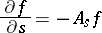(1)

(the inverse, backward or first, equation;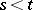), or of the form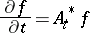(2)

(the direct, forward or second, equation;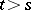), for the transition function,,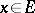,,being a measurable space, or its density, if it exists. For the transition function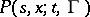the condition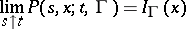is adjoined to equation (1), and the condition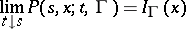is adjoined to equation (2), where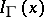is the indicator function of the set; in this case the operator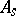is an operator acting in a function space, while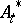acts in a space of generalized measures.

For a Markov process with a countable set of states, the transition function is completely determined by the transition probabilities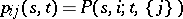(from the stateat instantto the stateat instant), for which the backward and forward Kolmogorov equations have (under certain extra assumptions) the form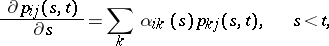(3)(4)

where(5)

In the case of a finite number of states, equations (3) and (4) hold, provided that the limits in (5) exist.

Another important class of processes for which the question of the validity of equations (1) and (2) has been studied in detail is the class of processes of diffusion type. These are defined by the condition that their transition functions,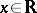,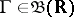, satisfy the following conditions:

a) for eachand,uniformly in,;

b) there exist functionsand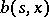such that for everyand,uniformly in,. If the density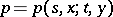exists, then (under certain extra assumptions) the forward equationholds (forand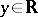) (also called the Fokker–Planck equation), while the backward equation (forand) has the form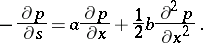How to Cite This Entry:
Kolmogorov equation. Encyclopedia of Mathematics. URL: http://encyclopediaofmath.org/index.php?title=Kolmogorov_equation&oldid=12711
This article was adapted from an original article by A.N. Shiryaev (originator), which appeared in Encyclopedia of Mathematics - ISBN 1402006098. See original article